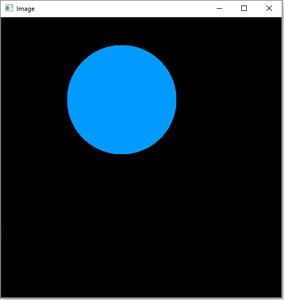Open in App
Not now

# Python OpenCV | cv2.circle() method

• Difficulty Level : Easy
• Last Updated : 04 Jan, 2023

OpenCV-Python is a library of Python bindings designed to solve computer vision problems. cv2.circle() method is used to draw a circle on any image. The syntax of cv2.circle() method is:

Syntax:

`cv2.circle(image, center_coordinates, radius, color, thickness)`

Parameters:

• image: It is the image on which the circle is to be drawn.
• center_coordinates: It is the center coordinates of the circle. The coordinates are represented as tuples of two values i.e. (X coordinate value, Y coordinate value).
• color: It is the color of the borderline of a circle to be drawn. For BGR, we pass a tuple. eg: (255, 0, 0) for blue color.
• thickness: It is the thickness of the circle border line in px. Thickness of -1 px will fill the circle shape by the specified color.

Return Value: It returns an image.

The steps to create a circle on an image are:

2. Pass this image to the cv2.circle() method along with other parameters such as center_coordinates, radius, color and thickness.
3. Display the image using cv2.imshow() method.

Implementation:

Image used for all the below examples:Example #1:

## Python3

 `# Python program to explain cv2.circle() method  ` `   `  `# importing cv2  ` `import` `cv2  ` `   `  `# path  ` `path ``=` `r``'C:\Users\Rajnish\Desktop\geeksforgeeks\geeks.png'` `   `  `# Reading an image in default mode ` `image ``=` `cv2.imread(path) ` `   `  `# Window name in which image is displayed ` `window_name ``=` `'Image'` `  `  `# Center coordinates ` `center_coordinates ``=` `(``120``, ``50``) ` ` `  `# Radius of circle ` `radius ``=` `20` `  `  `# Blue color in BGR ` `color ``=` `(``255``, ``0``, ``0``) ` `  `  `# Line thickness of 2 px ` `thickness ``=` `2` `  `  `# Using cv2.circle() method ` `# Draw a circle with blue line borders of thickness of 2 px ` `image ``=` `cv2.circle(image, center_coordinates, radius, color, thickness) ` `  `  `# Displaying the image  ` `cv2.imshow(window_name, image)  `

Output:Example #2: Using thickness of -1 px to fill the circle with red color.

## Python3

 `# Python program to explain cv2.circle() method  ` `   `  `# importing cv2  ` `import` `cv2  ` `   `  `# path  ` `path ``=` `r``'C:\Users\Rajnish\Desktop\geeksforgeeks\geeks.png'` `   `  `# Reading an image in default mode ` `image ``=` `cv2.imread(path) ` `   `  `# Window name in which image is displayed ` `window_name ``=` `'Image'` `  `  `# Center coordinates ` `center_coordinates ``=` `(``120``, ``100``) ` ` `  `# Radius of circle ` `radius ``=` `30` `  `  `# Red color in BGR ` `color ``=` `(``0``, ``0``, ``255``) ` `  `  `# Line thickness of -1 px ` `thickness ``=` `-``1` `  `  `# Using cv2.circle() method ` `# Draw a circle of red color of thickness -1 px ` `image ``=` `cv2.circle(image, center_coordinates, radius, color, thickness) ` `  `  `# Displaying the image  ` `cv2.imshow(window_name, image)  ` ` `

Output:Example # 3: To display a circle on a black screen created using NumPy library.

## Python3

 `import` `cv2 ` `import` `numpy as np ` `    `  ` `  `# Reading an image in default mode ` `Img ``=` `np.zeros((``512``, ``512``, ``3``), np.uint8) ` `    `  `# Window name in which image is displayed ` `window_name ``=` `'Image'` `   `  `# Center coordinates ` `center_coordinates ``=` `(``220``, ``150``) ` `  `  `# Radius of circle ` `radius ``=` `100` `   `  `# Red color in BGR ` `color ``=` `(``255``, ``133``, ``233``) ` `   `  `# Line thickness of -1 px ` `thickness ``=` `-``1` `   `  `# Using cv2.circle() method ` `# Draw a circle of red color of thickness -1 px ` `image ``=` `cv2.circle(Img, center_coordinates, radius, color, thickness) ` `   `  `# Displaying the image ` `cv2.imshow(window_name, image) ` `cv2.waitKey(``0``) ` `cv2.destroyAllWindows()`

Output:Circle on Black background

My Personal Notes arrow_drop_up
Related Articles